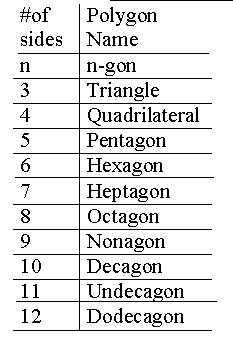Basic Polygon Names and Formulas
 Polygon Formulas - Area of regualr polygon - 1/2 x (apothem*) x (perimeter)Sum of the interior angles of a polygon - (number of sides -2) x (180)Number of diagonals in a polygon- (1/2 # of sides) x (number of sides)All the exterior angles of every polygon add up to 360 degrees